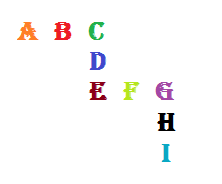# Equal sums

Logic Level 2Each of the digits 1,2,3,4,5,6,7,8, and 9 is represented by a different letter in the figure above.

They are positioned in such a way, that the sum of each row or column of the letters is equal to 13.

Which digit does 'E' represents❓

Also try Equal Products

×